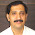### Instrument Voltage Transformer

Instrument Transformer and Power Management (P1) Course
Chapter (7) : Instrument Voltage Transformer
7.1 General purpose voltage transformer
7.1.1 Instrument Voltage Transformer
The voltage transformer is a transformer intended for measuring or control purposes, which is designed to have its primary winding connected in parallel with a circuit, the voltage of which is to measured or controlled.
The voltage transformer transforms a high voltage to standard value., usually 115 V. In this way, standards instrument and relays can be used and the worker is protected against dangerously high voltages.Voltage transformers are also used for isolation purposes and in metering circuits they are used to provide a voltage of a polarity reversed to that of the power circuit. Ratios range from one to 115 to 550.000 V.

7.2 Principles of operation :
In general, the secondary voltage is proportional to ratio of turns and opposite in phase to the primary voltage. This relationship is not completely exact for the following reasons :
a) The exciting current that necessary to magnetize, the iron core cause an impedance drop in the primary winding.
b) The load current that is drawn by the burden causes an impedance drop in both the primary and secondary windIngs.

Both of these produce an overall voltage drop in the transformer and introduce errors in both ratio and phase angle. The net result is that the secondary voltage is slightly less than the ratio of turns would indicate and there is a slight shift in the phase relationship.

The tow errors are called the ratio error and the phase angle error. These errors are shown graphically in Fig. (1) and Fig. (2) for a one to one ratio transformer.

Ep = Es + Is (Rs + JXs) + Ip (Rp + JXp)
Es = Ep - Is (Rs + JXs) - Ip (Rp + JXp)

The following is the legend for Fig. (2) and Fig. (3)

Rb = Resistance of buredn.
Xb = Reactance of burden.
Es = Secondary terminal voltage.
Is = Secondary current.
θs = Angle of lag of secondary.

Is Rs = E.M.F consumed in the secondary winding by the resistance.
Is Xs = E.M.F consumed in the secondary winding by the reactance.
E0 = Is Zs + Es = E.M.F induced in secondary winding.
θ = Flux required to induce the E.M.F .EG.
Im = Primary current required to the flux.
ICL = Primary current required to supply core loss.
IE = Im + ICL = Total current necessary to excite the core.
E = Angle of Exciting current . IF.
Is = Current in the primary necessary to balance, magnetically. The secondary current Is.
Ip = IE + (- Is) = Total primary current.
Eg = Voltage required to overcome the E.M.F induced in the primary winding (induced primary voltage).
IpRp = E.M.F  consumed in the primary winding by resistance.
IpXp = E.M.F consumed in the primary winding by reactance.
IpZp = Total E.M.F consumed in the primary winding.
Ep = IpZp + ( -Eg) = Impressed voltage (primary terminal voltage).
Es = Secondary terminal voltage.
= Phase angle of the transformer = Angle between primary voltage and reversed secondary.Fig. (1)Fig. (2)
1.